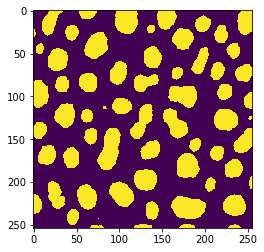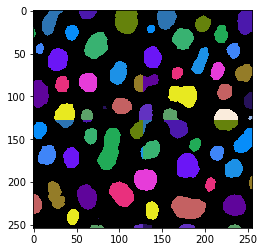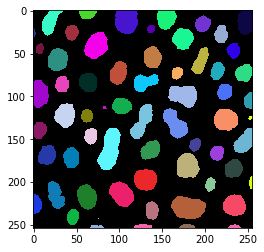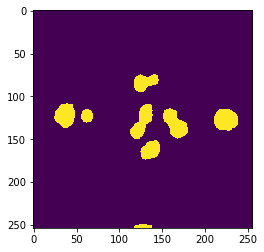# Connected component labeling in tiles#

Labeling objects in tiles is a challenging task. If this step cannot be circumvented, the dask-image offers the right functionality to retrieve label images with labels crossing multiple tiles.

import numpy as np
from skimage.data import cells3d
from skimage.measure import label as skimage_label
import pyclesperanto_prototype as cle
from pyclesperanto_prototype import imshow

image = imread("../../data/blobs.tif") > 128
imshow(image)tiles = da.from_array(image, chunks=(128, 128))
tiles

 Array Chunk 63.50 kiB 16.00 kiB (254, 256) (128, 128) 4 Tasks 4 Chunks bool numpy.ndarray

We will use scikit-image function label() for processing our image.

procedure = skimage_label

tile_map = da.map_blocks(procedure, tiles)

result = tile_map.compute()
imshow(result, labels=True)In this image, we can already see that the result has artifacts at the tile borders in the horizontal and vertical image center. To further check the result, we will compute the connected component labeling of the image without tiling.

reference = skimage_label(image)

imshow(reference, labels=True)Next, we can compare the maximum intensity in the label image to see if the total number of labels in these two images are equal. They are not:

result.max(), reference.max()

(20, 63)


By computing the standard deviation of the pixel intensity in the result image grouped by the reference labels, we can visualize which objects are not segmented correctly.

stddev_map = cle.standard_deviation_intensity_map(result, reference) > 0

imshow(stddev_map)## Tiled connected component labeling using dask-image#

The image processing library dask-image has a distributed version of connected component labeling available dask_image.ndmeasure.label:

result_di, num_labels = daskimage_label(image)

imshow(result_di, labels=True)Note that the result of this operation is a dask-array, and computing the maximum for example is not straightforward:

result_di.max(), reference.max()

(dask.array<amax-aggregate, shape=(), dtype=int32, chunksize=(), chunktype=numpy.ndarray>,
63)

np.asarray(result_di).max(), reference.max()

(63, 63)